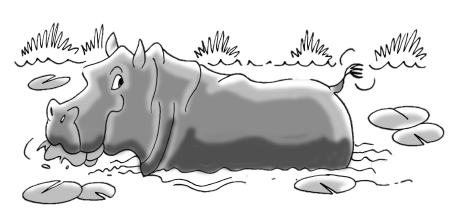### Home > CC3 > Chapter 6 > Lesson 6.1.1 > Problem6-7

6-7.

Homer the Hungry Hippo is munching on the lily pads in his pond. When he got to the pond, there were $30$ lily pads, but he is eating $5$ lily pads an hour. Henrietta the Hungrier Hippo found a better pond with $38$ lily pads! She eats $7$ lily pads every hour.1. If Homer and Henrietta start eating at the same time, when will their ponds have the same number of lily pads remaining?

Write an expression to represent the number of lily pads in Homer's pond. Write another expression for Henrietta's pond.

Set the expressions equal to each other and solve for $h$ (hours).

Subtract $30$ from both sides.

Add $7h$ to both sides.

Divide both sides by $2$.

$h=4$ hours

Homer: $30−5h$
Henrietta: $38−7h$

$30−5h=38−7h$

$−30−30$
$−5h=8−7h$

$+7h+7h$
$2h=8$

$h=\frac{8}{2}$

2. How many lily pads will be left in each pond at that time?

Use the expressions you wrote in part (a).

Substitute the answer for h into the expressions.

Homer:
$30−5h$
$30−5(4)$
$10$

Henrietta:
Repeat the process.

$10$ lily pads